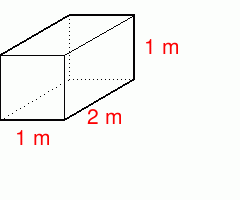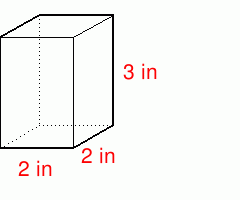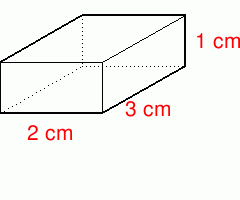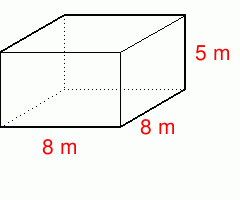MathScore EduFighter is one of the best math games on the Internet today. You can start playing for free!

## Rectangular Solids - Sample Math Practice Problems

The math problems below can be generated by MathScore.com, a math practice program for schools and individual families. References to complexity and mode refer to the overall difficulty of the problems as they appear in the main program. In the main program, all problems are automatically graded and the difficulty adapts dynamically based on performance. Answers to these sample questions appear at the bottom of the page. This page does not grade your responses.

Want unlimited math worksheets? Learn more about our online math practice software.
See some of our other supported math practice problems.

### Complexity=1

Find the surface area and volume. Answer with proper units. To represent m2, use "sq m". Sample answer: "5 sq m". To represent m3, use "cu m".

 1surface area = volume = 2surface area = volume =

### Complexity=2

Find the surface area and volume. Answer with proper units. To represent m2, use "sq m". Sample answer: "5 sq m". To represent m3, use "cu m".

 1surface area = volume = 2surface area = volume =

## Answers

### Complexity=1

Find the surface area and volume. Answer with proper units. To represent m2, use "sq m". Sample answer: "5 sq m". To represent m3, use "cu m".

#ProblemCorrect AnswerYour Answer
1surface area =
volume =
Solution
The surface area is the area of the six sides. This is twice the area of the three different kinds of sides.
= 2 × ((2m × 1m) + (1m × 1m) + (1m × 2m)) = 10 m2.
V=lwh (length × width × height)
= 1m × 2m × 1m = 2 m3.
#ProblemCorrect AnswerYour Answer
2surface area =
volume =
Solution
The surface area is the area of the six sides. This is twice the area of the three different kinds of sides.
= 2 × ((2in × 3in) + (3in × 2in) + (2in × 2in)) = 32 in2.
V=lwh (length × width × height)
= 2in × 2in × 3in = 12 in3.

### Complexity=2

Find the surface area and volume. Answer with proper units. To represent m2, use "sq m". Sample answer: "5 sq m". To represent m3, use "cu m".

#ProblemCorrect AnswerYour Answer
1surface area =
volume =
Solution
The surface area is the area of the six sides. This is twice the area of the three different kinds of sides.
= 2 × ((3cm × 1cm) + (1cm × 2cm) + (2cm × 3cm)) = 22 cm2.
V=lwh (length × width × height)
= 2cm × 3cm × 1cm = 6 cm3.
#ProblemCorrect AnswerYour Answer
2surface area =
volume =
Solution
The surface area is the area of the six sides. This is twice the area of the three different kinds of sides.
= 2 × ((8m × 5m) + (5m × 8m) + (8m × 8m)) = 288 m2.
V=lwh (length × width × height)
= 8m × 8m × 5m = 320 m3.
Learn more about our online math practice software."MathScore works." - John Cradler, Educational Technology Expert© Copyright 2010 Accurate Learning Systems Corp. All rights reserved.